Zeng, J.-S., Chen, K.-F., and Li, J. (2011). "Study of the bulk density of high consistency pulp and engineering application in the bleaching tower," BioRes. 6(1), 594-605.

#### Abstract

From experimental simulation of the process of high consistency pulp moving in a bleaching tower, the aerated bulk density and packed bulk density were measured and studied by using a self-made experimental system. The scattered experimental data – pressure p, and bulk density difference, which was between packed bulk density and aerated bulk density (ρ-ρ0) – were fitted by using Matlab software, and some good-fitting regression curves and equations were obtained. The results showed there was a break point W in the regression curves; within the range 0 £ p £ W the relationship between (ρ-ρ0) and p was a linear function, while for W £ p £ 70000 the relationship was a power function. To effectively meet with the bleaching response for the different kinds and different consistencies of pulp in the tower, by using the fitting regression equations combined with the expressions of average bulk density and pressure in the tower caused by gravity-driven pulp, two equations for average packed density ρa were deduced with the aim of deciding the maximum volume value of the tower, in agreement with the sizes of the towers presently used by major companies.

STUDY ON THE BULK DENSITY OF HIGH CONSISTENCY PULP AND ENGINEERING APPLICATION IN THE BLEACHING TOWER

Jin-Song Zeng,a Ke-Fu Chen,a and Jun Li a,*

From experimental simulation of the process of high consistency pulp moving in a bleaching tower, the aerated bulk density and packed bulk density were measured and studied by using a self-made experimental system. The scattered experimental data – pressure p, and bulk density difference, which was between packed bulk density and aerated bulk density (ρ-ρ0) – were fitted by using Matlab software, and some good-fitting regression curves and equations were obtained. The results showed there was a break point W in the regression curves; within the range 0 p W the relationship between (ρ-ρ0) and p was a linear function, while for W p 70000 the relationship was a power function. To effectively meet with the bleaching response for the different kinds and different consistencies of pulp in the tower, by using the fitting regression equations combined with the expressions of average bulk density and pressure in the tower caused by gravity-driven pulp, two equations for average packed density ρa were deduced with the aim of deciding the maximum volume value of the tower, in agreement with the sizes of the towers presently used by major companies.

Keywords: High consistency pulp; Aerated bulk density; Packed bulk density; Regression equation

Contact information: a: State Key Laboratory of Pulp and Paper Engineering, South China University of Technology, Guangzhou, 510640, China.

*Corresponding author: zjs789zjs@126.com; zjs789zjs@yeah.net

INTRODUCTION

Due to their effectiveness in mass transfer, high consistency towers are widely used as the reactors for pulp delignification or bleaching. As a consequence, high consistency pulp bleaching towers are well established as key pieces of equipment in high consistency bleaching production. But so far, published studies have not yet estab-lished a suitable equation for integrated engineering calculations of the tower.

Calculations to express the volume and diameter for the tower have been introduced (Chen 2005), but the key variables underlying the calculations, such as the bulk density, have not been adequately defined. In the Light Industry Handbook, the expression for the bulk density and some coefficients for different kinds of pulp have been simply provided (1995), but it is well known that the bulk density is greatly influenced by water content or consistency of pulp, which is unfortunately not taken into account in the expression. Up until the present moment, there has been no appropriate way to decide the bulk density of the pulp.

To satisfy the requirements of the engineering calculations for the volume and diameter for the tower, there are two kinds of bulk densities to be investigated: aerated bulk density and packed bulk density. The aerated bulk density of pulp is determined by allowing the dispersed pulp to settle under free fall into a container, and the packed bulk density is determined by imposing a controlled pressure on the aerated sample. Some studies concerning aerated bulk density and packed bulk density of powder, soil, and distillers dried grains with solubles (DDGS) have been reported: The rate of densification between the aerated and tapped states has been used to provide an additional descriptor of the bulk structure and the micro- structure of the powder (Mohammadi 1997). A kind of correcting bulk density measurement has been made with driving hammer equipment for investigations of changes of soil carbon with time (Parfitt 2010). The aerated bulk density variability of DDGS during filling of railcars hoppers has been investigated (Clementson 2010). And some researchers have explored the use of a density bottle to measure bamboo fiber density (Sun, et al. 2007). Liquid buoyancy has been used to measure the density of raw wool fiber and to analyze the influences of different fiber structure on the measured density values (Dong, et al. 2009). As stated previously, the bulk density is quite different from the density of the material itself. As far as the powder, soil, and DDGS are concerned, the measurements of the aerated and tapped bulk density have become fairly mature. However, studies on measurement and determination of pulp fiber bulk density are still very limited. Pulp contains fibers of different sizes. Under typical conditions of accumulation, the bulk density of high consistency pulp is closely related to the kind of pulp fiber and the pressure on it. Therefore, the primary objective of this study was to simulate downward movement of pulp in a high consistency bleaching tower (HC tower), and determine the relationship between the gravity-driven tower pressure p and the bulk density difference (ρ-ρ0). Meanwhile, by means of the relationships, the two equations for average packed density ρato decide the maximal volume value of the tower were deduced to be able to meet the needs of engineering calculations, in agreement with the sizes of the present towers that are in use in major companies.

EXPERIMENTAL

Usually the consistency of a high consistency pulp is in the range of 28% to 32%. Accordingly, the range considered in the present study was 24% to 35%. As shown in Fig. 1, after the pulp enters the tower from the top, the packed density gradually increases with the accumulation of pulp, which in the present case was CTMP. If its packed density is ρ at position 1, the gravity-driven pressure caused by the pulp is P, and then its packed density is ρ+ Δρ at position 2, where the pressure is P + ΔP. This means that the packed bulk density is closely related to the pressure at different positions, and the two values will be the greatest on the surface of the discharging zone at the bottom of the tower.

Materials and Equipment

The pulp sample was obtained from the medium and high consistency bleaching production line at the State Key Laboratory of Pulp and Paper Engineering, South China University of Technology. As shown in Fig. 2, after the pulp was adjusted by the two-spin thickener to the high consistency level, the pulp was introduced into the high consistency mixer via the pulp inlet at the top of the mixer, in which it was sheared with high speed to become very loose and consequently well mixed with bleaching agent.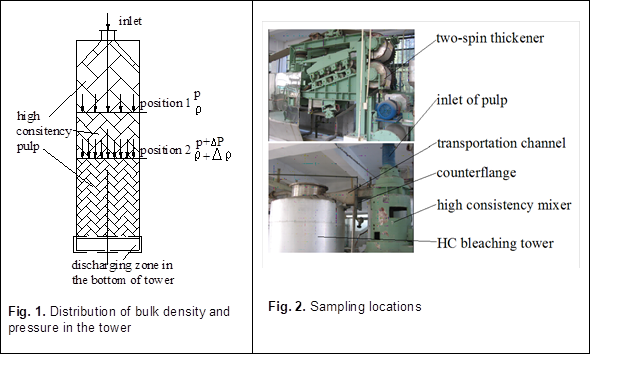Then it passed into the HC bleaching tower from a tangential direction by way of a transportation channel. To measure the bulk density, the pulp can be sampled in a counterflange before the transportation channel, and later to be put into the clear plastic tower to be ready for experimentation.

Aerated Bulk Density Measurement

Based on the distribution of bulk density and pressure in the tower, after the pulp was sampled, the following experiment was designed. As shown in Fig. 3, in the experiment, it was made up of the following parts: a clear plastic tower made from poly-(methylmethacrylate) (PMMA) (mass: 5kg, sectional area: 0.05 m2), a pillar weighing cell (type: MCC DBST), a weighing transmitter (range: 0 to 500 mm, accuracy: 0.5%), a cover plate made of wood, PLC (Siemens S7-200), KTF300 linear displacement transducer (UNCAN CO.LTD), and a portable computer.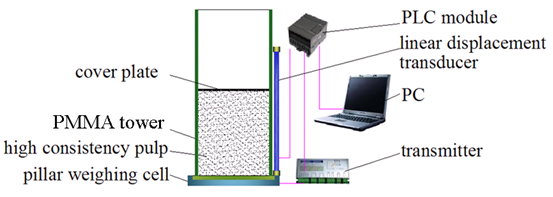Fig. 3. Experimental scheme for aerated bulk density

The pulp sample was piled up in the fixed PMMA tower, and prepared for the experiment after being made flat. The cover plate was placed on the flat surface of the high consistency pulp; the cover plate was so light that it could not cause the pulp to move on account of the bridge formed among the pulp fibers (Xuan 2007). A linear displacement transducer was placed on the side wall of the PMMA tower; its press plate was attached to the cover plate, and thus a 4 to 20 mA standard signal of displacement h was sent to a PLC analogue module (Zhang 2007). In addition, the total weight m1 of the pulp and PMMA tower, and the weight m2 of the PMMA tower were also sent to PLC module by way of the pillar weighing cell and transmitter, respectively. A personal computer was set up to communicate with the PLC module. Thus the values of weight and volume were obtained to determine the bulk density. The values of the bulk densities themselves were not useful as absolute quantities, but they were used as sensitive five-times repeatable measurement to identify any slight changes in the morphology of the primary fiber particles and their movement characteristics during the process.

All data points were processed in the upper monitor (PC) after being processed by the PLC module according to the following equation,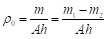(1)

where o is the bulk density (kg/m3), m is weight of pulp (kg), and m1 is weight of pulp and PMMA tower (kg ), mis weight of PMMA tower(kg ), A is section area of PMMA tower (m2), and is the height of pulp in the PMMA tower(m).

Packed Bulk Density Measurement

A pressurizing system (Vanremortel 1993; Stone 1991) was added to measure the packed density by giving a controlled pressure to the aerated sample. The system (provided by Shenzhen hydraulic components Co., Ltd.) with steady pressure and controlled speed consisted of a hydro-cylinder, a speed-regulating valve, an overflow valve, an electro-connecting pressure gauge, a check valve, a three-position five-way valve, and an oil pump, as shown in Fig. 4. After the pressure of hydraulic liquid was set by the overflow valve and electro-connecting pressure gauge, the downwards speed of piston was dependent on the speed regulating valve, so the hydraulic liquid could be used to drive the piston with constant pressure and speed for the high consistency pulp. The pulp would tend to lag behind the piston to move down for a few minutes, the cover plate and press plate of the linear displacement transducer would then move, and subsequently the displacement h signal was sent to PLC module by the linear displacement transducer with transmitter. Meanwhile, the weight signal m1g (where m1 is given in the measurement of aerated bulk density) was sent to the transmitter by the pillar weighing cell, and later to the PLC module with the 4 to 20 mA standard signal resulting from the transmitter.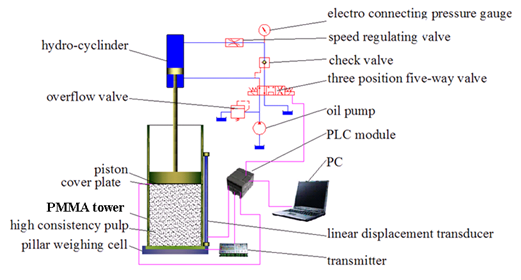Fig. 4. Schematic diagram for experiment of packed density

During each measurement, the piston, cover plate, and press plate of the linear displacement transducer stayed close together to add to the pressure on the pulp by means of pressurizing system. The pressure shown on the electro-connecting pressure gauge was controlled by adjusting the three-position five-way valve and oil pump. In the experiment, the speed when the hydro-cylinder was driving the piston was the same as the descending rate of the pulp in the tower, about 3mm/s, which was decided by the height of tower and reaction time, and would affect the packing density of the pulp. So the movement was slow and continuous. All terminal data was processed in the upper monitor (PC) after being communicated with PLC module according to the following equations,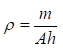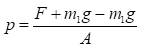(2)

where is the packed density (kg/m3), p is the pressure (Pa), and F is the applied force (N). A and m were parameters identical to those used for the aerated bulk density. But the bigger the pressure on the hydro-cylinder, the smaller was the value of h. It is important to note that the pressure produced by the pulp itself was negligible, compared to the pressure on the pulp exerted by the hydro-cylinder in the above-mentioned “p” expression (Eq. 2).

RESULTS AND DISCUSSION

In the experiment, Masson pine CTMP pulp mixed with bleaching agent was put into the PMMA tower to test the aerated and packed bulk density. Later, discrete data, such as the pressure p, packed bulk density , and aerated bulk density o were obtained from the PC of the experiments. As shown in Table 1, when the pressure was zero, the corresponding bulk density was the aerated bulk density o; when the pressure was not zero, the corresponding bulk density was the packed bulk density . Meanwhile the data showed that whenever the consistency was increased, the bulk density was reduced under the same pressure conditions. But when the pressure was increased the packed bulk density increased as well. When the pulp was subjected to the greatest pressure at 70,000 Pa, the highest bulk density (0.7277×103 kg·m-3) was attained at the lowest consistency pulp (24%, mass based). However, the minimum aerated bulk density was 0.1786 x103 kg·m-3, resulting from the highest consistency pulp at zero pressure condition, and hence the loosest structure.

By using the original scattered data from Table 1, the difference between aerated bulk density and packed bulk density (ρ-ρ0), was plotted against the pressure for the Masson pine pulp with different consistency. To clearly indicate the relationships, results were plotted for the ranges 0 to 7500 Pa and 7500 to 70,000 Pa, as shown in Figs. 5 and 6. According to the trend of the discrete data points, MATLAB can be adopted to complete the model fitting. The fitted regression curve showed a good linear relation between (ρ-ρ0) and p when the pressure was less than 7500Pa, while there was good power function between them when the pressure was more than 7500Pa. 7500Pa can be considered as a boundary point between the subsection functions, as shown in Figs. 5 and 6.

Table 1. Bulk Density of Masson Pine CTMP Pulp at Different Pressure (x103kg·m-3)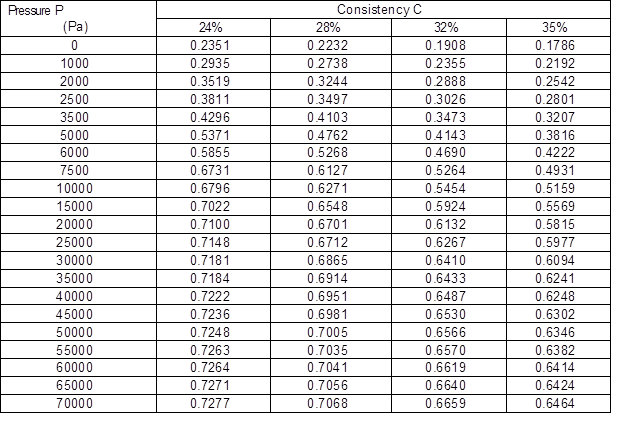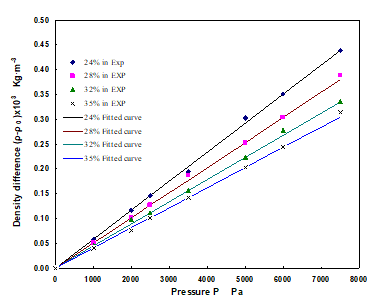Fig. 5. Relationships between density difference and pressure for Masson pine CTMP pulp within a scope of 0 to 7500 Pa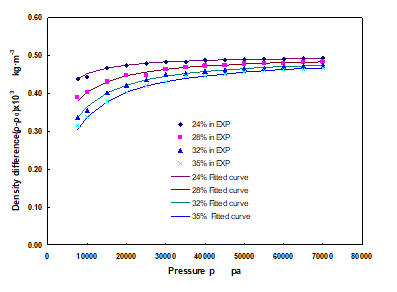Fig. 6. Relationships between density difference and pressure for Masson pine CTMP pulp over 7500 Pa

Table 2 shows the bulk density model fitting of the regression curves from Figs. 5 and 6, which gives a useful quantitative analysis. From the regression equation, it shows that the packed bulk density was significantly related to the consistency, pressure, and aerated bulk density, which was closely related to the kinds of pulp. Meanwhile, the correlation coefficient of fitted equation was more than 0.97, so the fitted curves were in good agreement with the diverse data.

Table 2. Regression Equations for Different Consistency for Masson Pine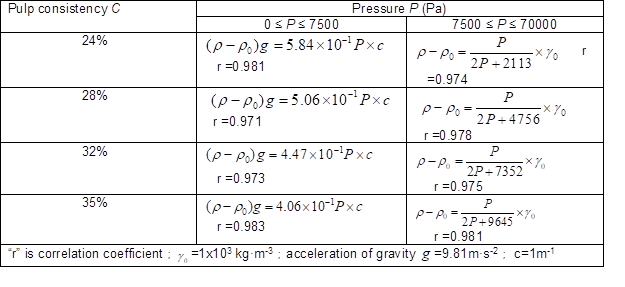CTMP Pulp

Based on the results of experiments carried out with bamboo, mulberry, and bagasse pulp, it can be concluded that there are similar function relationships for most of the common kinds of pulp; however, the break points and coefficients of fitted regression curves were different, as shown in Fig. 7. These differences can be attributed to the gap between fibers and to the fiber characteristics. When the pressure is larger than the break point, the relations between (-o) and p can be a power function; when the pressure is smaller than the break point, a linear function is expected. The transitional pressure of bamboo, mulberry, and bagasse pulps were 8500 Pa, 7500 Pa, and 7000 Pa, respectively. The slope for the linear functions in the regression curves for the different kinds of pulp with same consistency were very different, the greatest for bagasse pulp, and the smallest for bamboo pulp.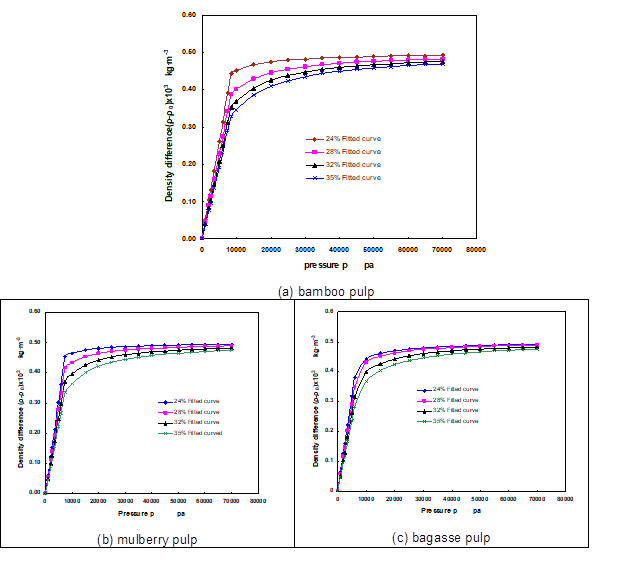Fig. 7. Fitted regression curves of different pulps

REGRESSION EQUATIONS IN THE ENGINEERING APPLICATION OF THE BLEACHING TOWER

From the experimental results for the types of pulp considered, the following conclusion can be made: When the difference o, and the pressure correspondingly are plotted for each consistency of the pulp, there will be a break point of data in the regression curve, and the relationships can be expressed as: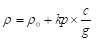for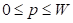(3)

and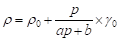for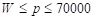(4)

The values of Woka, and are dependent on the kinds and consistencies of pulp, where is the pressure (Pa) at the transition point, is the linear coefficient (1/ Pa), is a coefficient of the power function (with no units), b is a coefficient of the power function (with units of Pa), and P is the pressure (Pa).

The average bulk density in the tower can be expressed as: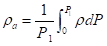(5)

Meanwhile, the pressure in the tower is: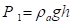(6)

Combined equations (3) through (6), the following expression is obtained: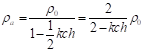for 0≤P≤W， (7)

and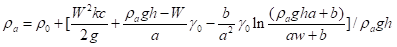W≤P≤70000 (8)

From equation (7) we can clearly conclude that if a variety of pulp with a smaller value and higher consistency is chosen for the calculation, a smaller a value will be attained.

However, from equation (8) it is difficult to determine values of the average packed bulk density ρa directly, so ρa , ρ0, and the height of material h can be plotted to represent the condition where the pressure is more than W, as shown in Fig. 8: if the aerated bulk density ρ0 is constant, the average packed density ρa will increase with increase of the height of material h. In particular when h is more than 10 meters, the value of ρa will increase greatly. However, a smaller ρa value will be obtained only when smaller values of h and ρare chosen.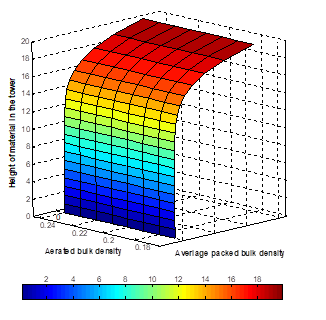Fig. 8. Relation of bulk density and material level when the pressure P is over W

For engineering calculations, the volume of the bleaching tower can be expressed as follows (Chen 2005):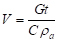(9)

where V is the volume of the bleaching tower (m3), G is the production capacity (kg/s), t is the reaction time in the tower (s), a is the average compacted density (kg/m3), and C is the consistency of the pulp (%).

From equation (9), in order to meet the requirements for all kinds of bleaching and reaction operations, the volume must be at its maximum value when the production capacity and reaction time in the tower are constant, so that Ca is at a minimum. The range of pulp consistency C is within the range 24% to 35%; however, the value of a is only derived from the equations (7) and (8). Although it can be obtained qualitatively from equations (7) and (8), in addition to Fig. 8, it cannot be calculated the Ca value directly. In order to obtain the minimum value of Ca quickly, ρ0c, k, g, h, a, b, W, and consistency of pulp C will be input and calculated by means of the program from equations (7) and (8) in the Matlab toolbox (Xiao 2001; Wang 2006). Thus the maximum volume value will be obtained. /in comparison with the present towers produced by major firms, the equations differ by 8% from both of the sets of results from the big companies Metso and Andritz.

CONCLUSION

When considering high consistency pulp moving in a high consistency bleaching tower, experiments were undertaken to clarify the relationship between pressure and bulk density. The results revealed:

(1) For most of common kinds of pulp, when the difference between the aerated bulk density and packed bulk density is plotted and fitted against the pressure, a break point can be observed in the data of the fitted regression curves. For 0pW, the relationship between (o) and p can be expressed as a linear function; while for Wp70000, the relationship can be expressed as a power function.

(2) The fitted regression equation for (o) and p, combined with average density and pressure in the tower, can be used to deduce an equation for ρa to determine the maximum volume value of the tower, and the equation can be useful and feasible in the engineering. In particular, the equation can be used to calculate the volume of the tower, in agreement with the sizes of present towers produced by the major suppliers of this type of equipment.

ACKNOWLEDGMENTS

The authors thank Ke-Fu Chen academician for critical reading of the manuscript. This work was supported by the grants from the National Natural Science Foundation of China (No.2006BAD32B06-2).

REFERENCE CITED

Chen, K. F. (2005). Bleaching Technique of High Consistency Pulp in Pulp and Paper Machinery and Equipment, Light Industry Publisher, Beijing.

Clemenston, K. L., and Ileleji, K. E. (2010). “Variability of bulk density of distillers dried grains with solubles (DDGS) during gravity-driven discharger,” Bioresource Technology 101, 5459-5468.

Dong, L., Zhang, J. F., and Xu, L. (2009). “Liquid buoyancy method to measure the density of raw wool fiber,” Knitting Industry 4, 62-66.

Light, I. A. (1995). Handbook of Equipment for Light Industry, 1st Ed., Mechanical Industry Publisher, Beijing.

Mohammadi, M. S. (1997). “Bulk density modeling as a means of typifying the microstructure and flow characteristics of cohesive powders,” Powder Technology 92, 1-8.

Parfitt, R. L. (2010). “Correcting bulk density measurements made with driving hammer equipment,” Geoderma 157, 46-50

Stone, J. A. (1991). “Core sampling technique for bulk density and porosity determination on a clay loam soil,” Soil Tillage Res. 21, 377-383.

Sun, Z. W., Yang, J. P., Yu, C. W. (2007). “Bamboo fiber density,” Science and Development of Textile 1, 75-76.

Vanremortel, R. D. (1993). “Comparison of clod and core methods for determination of soil bulk density,” Commun. Soil Sci. Plant. Anal. 24, 2517-2528.

Xuan, Z. N. (2007). “Voidage measurement of the medium and high consistency pulp,” China Pulp & Paper Industry (31), 31-33.

Zhang, H. R. (2007). Sensor Technology, Beijing University of Aeronautics and Astronautics Publisher, Beijing, China.

Xiao, J. S. (2001). MATLAB and Scientific Calculation, Tsinghua University Publisher, Beijing.

Wang, H. J. (2006). Analysis of Mathematical Statistics and Matlab Engineering Data, Tsinghua University Publisher, Beijing.

Article submitted: August 17, 2010; Peer review completed: October 28, 2011; Revised article received and accepted: December 29, 2010; Published: January 4, 2011.